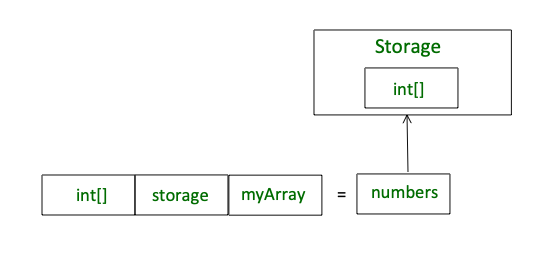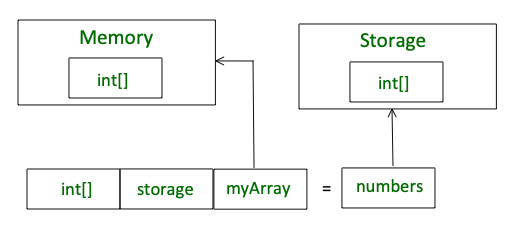Related Articles

# Storage vs Memory in Solidity

• Difficulty Level : Basic
• Last Updated : 16 Jun, 2021

Storage and Memory keywords in Solidity are analogous to Computer’s hard drive and Computer’s RAM. Much like RAM, Memory in Solidity is a temporary place to store data whereas Storage holds data between function calls. The Solidity Smart Contract can use any amount of memory during the execution but once the execution stops, the Memory is completely wiped off for the next execution. Whereas Storage on the other hand is persistent, each execution of the Smart contract has access to the data previously stored on the storage area.

Every transaction on Ethereum Virtual Machine costs us some amount of Gas. The lower the Gas consumption the better is your Solidity code. The Gas consumption of Memory is not very significant as compared to the gas consumption of Storage. Therefore, it is always better to use Memory for intermediate calculations and store the final result in Storage.

1. State variables and Local Variables of structs, array are always stored in storage by default.
2. Function arguments are in memory.
3. Whenever a new instance of an array is created using the keyword ‘memory’, a new copy of that variable is created. Changing the array value of the new instance does not affect the original array.

Example#1: In the below example, a contract is created to demonstrate the ‘storage’ keyword.

## Solidity

 `pragma solidity ^0.4.17;` `// Creating a contract``contract` `helloGeeks``{``  ``// Initialising array numbers``  ``int``[] ``public` `numbers;` `  ``// Function to insert values``  ``// in the array numbers``  ``function Numbers() ``public``  ``{``    ``numbers.push(1);``    ``numbers.push(2);` `    ``//Creating a new instance``    ``int``[] storage myArray = numbers;``    ` `    ``// Adding value to the``    ``// first index of the new Instance``    ``myArray = 0;``  ``} ``}`

#### Output:

When we retrieve the value of the array numbers in the above code, Note that the output of the array is [0,2] and not [1,2]

This can be understood from the following diagram –Using the keyword “storage”

Example#2: In the below example, a contract is created to demonstrate the keyword ‘memory’.

## Solidity

 `pragma solidity ^0.4.17;` `// Creating a contract``contract` `helloGeeks``{ ``  ``// Initialising array numbers``  ``int``[] ``public` `numbers;``  ` `  ``// Function to insert``  ``// values in the array``  ``// numbers``  ``function Numbers() ``public``  ``{``    ``numbers.push(1);``    ``numbers.push(2);``    ` `    ``//creating a new instance``    ``int``[] memory myArray = numbers;``    ` `    ``// Adding value to the first``    ``// index of the array myArray``    ``myArray = 0;``  ``} ``}`

Output:

When we retrieve the value of the array numbers in the above code, Note that the output of the array is [1,2]. In this case, changing the value of myArray does not affect the value in the array numbers.

This can be understood from the following diagram –Using the keyword “memory”

My Personal Notes arrow_drop_up